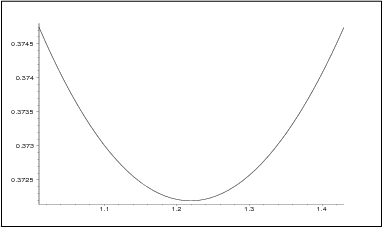]> 5.5 Conclusions

### 5.5 Conclusions

For a wide class of distributed parameter systems it is impossible or difficult to derive explicit formulae expressing the performance index as a function of the system parameters. In this case the proposed approximate methods lead to a numerical algorithm which is a reasonable compromise between simplicity of calculations, applicability of the standard software and accuracy of results. The main barrier in getting better accuracy is the ill–conditioning of the Hankel–Hilbert matrix.

In Matlab implementation, to overcome this barrier it is recommended to solve (5.3) using the command "$Z\setminus d$" specialized for solving linear algebraic equation $Zx=d$. On the basis of two examples presented in Section 5.4 we conclude that the accuracy may be sometimes insufficient for control engineering applications. To be more precise the accuracy may turn to be not enough to recover the performance index in the vicinity of a minimum of the performance index. To avoid such effects the variable point arithmetics, as offered by e.g. Maple 6, is recommended. Figure 5.4 shows the result of implementing (5.10) on Maple 6 with Digits:=90; command to get $n=32$–dimensional approximation of the performance index (5.11). The results are decidedly better than those presented in Figure 5.2.Figure 5.4: Plots of the performance index for the system examined in Example 1. Here a precise approximation was achieved by the variable–point arithmetics from Maple 6